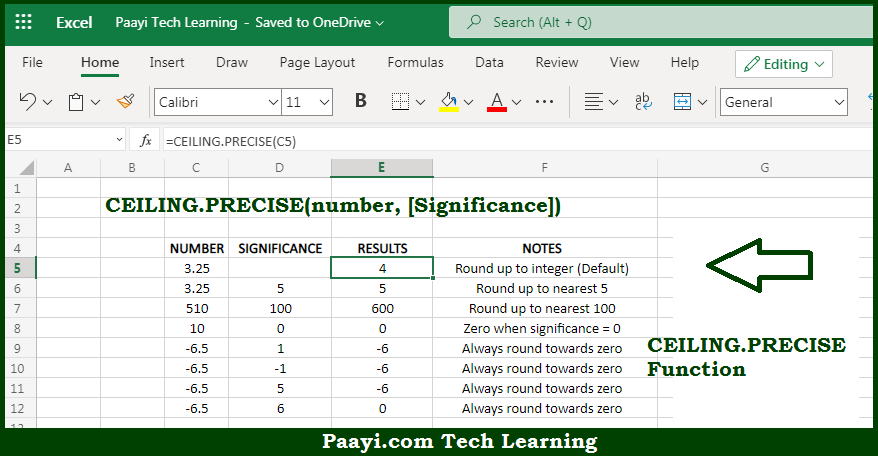# Learn How to Use Microsoft Excel CEILING.PRECISE Function

Written by | 0 Comments | 549 Views

In this article, you will learn how to use Microsoft Excel CEILING.PRECISE function and its prime function in Microsoft Excel. You will also get to know the Microsoft Excel CEILING.PRECISE function return value and syntax with the help of some examples.

Microsoft Excel CEILING.PRECISE Function

The main function of Microsoft Excel CEILING.PRECISE function is to round up a number to the nearest multiple. So, with the help of CEILING.PRECISE function, you can able to round up the number to the nearest specified multiple. The function CEILING.PRECISE function operates different from the CEILING function, as CEILING.PRECISE defaults to a multiple of 1, and always round negative numbers towards 0. So, with the help of Microsoft Excel CEILING.PRECISE function you can easily round up the number to the nearest multiple.

Return Value of CEILING.PRECISE Function

The return value will be the rounded number.

Syntax of CEILING.PRECISE Function

=CEILING.PRECISE(number, [significance])

Where the arguments:

• number: This is the number you want to round up.
• significance: This is the multiple used while rounding up the number, default is 1 (optional).

How to Use Microsoft Excel CEILING.PRECISE Function?Microsoft Excel CEILING.PRECISE function is used to round up a number up to a nearest integer or multiple of significance. By default, CEILING.PRECISE rounds to the nearest integer, using a significance of 1. Positive numbers with decimal portions are rounded up to the nearest integer and negative numbers with decimal portions are rounded toward zero. The CEILING.PRECISE is different from the CEILING function as, it defaults to a multiple of 1, which implies rounds to the nearest integer.

The CEILING.PRECISE always round negative numbers towards zero and CEILING.PRECISE ignores the sign of significance argument, which means uses the absolute value. So, with the help of Microsoft Excel CEILING.PRECISE function you can easily round up the number to the nearest multiple.